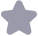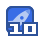### 论文研究-基于网结构压缩技术的Petri网监控器综合.pdf收藏

1054 系统工程理论与实践 第34卷 和(v,k)描述,那么这两个Peti网控制问题等价的含义为:从该离散事件系统的任何一个状态ⅹ开始 (X在这两个 Petri网系统中分别用m和m表示)两个网系统的不可控可达标识的最大加权和相等、即 max Ww. m' maX 设新网(N,mo)从任意标识m开始的不叮控可达标识集为:Bru(m)={m1,m2;……,mk}(k可以 是∞),根据新网与原网之间的关系,那么原网(N,mo)从任意标识m开始的不可控可达标识集划分如下 Ru(m)=R1∪R2∪…∪Rk(k可以是∞),R∩R=0,≠j并且Wm2∈R(1≤i≤k),m;∈ fu(m),v∈P-{mn}→m()=m2(D),m(mn)=m2(ma)+m(m)设mna∈R,(1≤j≤k), ·mna=ax:mn.根据上述R(m)的划分可知 m∈R vp∈P-{pn,(p)=v(p),并且m()=m),根据v(ma)≤s(m)和(1)式x(m)(1) m;∈R(m,vp∈P-{Pn}→m;(p) (p),mi(pr)=mmax(pa)+mI D·m7=3·mmax 根据(2)式,mem(m1m=m nax max max.m.证毕 0<1<k ′∈R 根据定理1构造网结构压缩的约束简化算法,见算法1. 算法1网结构压缩的约束简化算法 输人:(N,m0)和(m,k) 2. for all t∈Tvc∧|t°-|°t-1do 3.tx←t.令t的输入库所为pa,tx的输出库所为pb pa·∩Te={t}Aw(Pa)≤(Pb)t 5.将pa、tx和pb压缩为库所px,并且"pn=pa∪("pb-{tx}) 7.m0(n)=m0(Pn)+m0(Pb),c(mn)=(p) 8.将列向量mo的分量mo(Pa)删去,并且用mo(P)替换其分量m(P),其它分量不变 将行向量的分量w(a)删去,并且用v(x)替换其分量w(b),其它分量不变 p←pn,P←P∪{p tr 10.讠←i+1 11. endif 12. endfor 13.m0←m0,t←t 输出:(N,m0)和(v,k 定义1已知 Petri冈N=(P,,I,O,p∈P,设lb=(P",m,,O),如果满足下列条件: 1)P={p∈P存在从P到p’的不可控路径},Tv。={∈Tc存在从p到t的一条不可控路径}; 2)I={(D,+)(P,t)∈IA(P,∈Pxm},即I由I中那些两个结点均属于P×Tme的有向弧组成; O′={(t,p)(t,p)∈O∧(t,p)∈1l×P},即O由O中那些两个结点均属于mXP的有向弧组成则称 功为库所p的后向域 定理2给定一个Petm网控制问题,即在 Petri网系统(N,mo)施加线性约束(m,k),pa和pb是两个 库所,t是一个不可控变迁pa的后向域。是状态机,如果tn∈pa∩'pb,并且v(mb)=max{(p)}则 将pa、ta和Pb压缩为库所px,从而得到新网系统(N,m0)利新约束(,k),其中:P=P-{pa,pb}+{Px}, T=T-{t},T=-{(a,ta)}+{(x,t)},O=O-{(t2,mb)}+∪{(t,pn)} t∈ph·∪pa·-{ta} l(pn)=0(Pb),m0(P)=mo(Pa)+mo(Pb),并且vp∈P-{pn},m0(p)=m0(D),v(p)=w(p),那么新控制 叫题(即在(N,mo)上施加(ω,k)与原控制问题等价,即它们的最优监控器对实际被控对象的限制性相同 证明由两个 Petri网系统(N,mo)和(N,mo)描述的司一个离散事件系统,如果该离散事件系统的 个禁止状态控制目标可以分别用(N,m0)上的一个线性约束(,k)和(N,mo)上的一个线性约束(v,k)米 描述,那么这两个 Petri网控制问题等价的含义为:从该离散事件系统的任何一个状态X开始(X在这两个 Petri网系统中分别用m和m表示),两个网系统的不可控可达标识的最大加权和相等,即max”m′ m'∈Rrc(m) 第4期 罗继亮,等:基于网结构压缩技术的 Petri网监控器综合 1055 maX R uc(m) 设新网(N,mo)从任意标识m开始的不可控可达标识集为:R(m)={m1,m2,…,mk},(k可以 是∞),根据新网与原网之间的关系,那么原网(N,m)从任意标识m开始的不可控可达标识集划分如下 fu(m)=R1∪R2∪…∪Rk(k可以是∞),R∩;=0,≠j并且m∈R(1≤i≤k),彐m;∈ Ruc(m), pEP-px)=mi(p)=mx(p), mi(px)=mz(pa)+mx(pb 设ma∈R1(1≤j≤k),w·mnax=max{:m}.根据上述R(m)的划分可知: m;∈R(m),v∈P-{p}→m()=mmax(m),m:(P1)=max(Pa)+max(P) 根据状态机的性质,b。内任意不可控变迁序列的激发都不会使,内增加新的托肯CP-{pa (p)=(p),并且(px)=(pb),根据o(Pb)=max{(m)}和(3)式可知: Vp∈I 根据(4)式 nax l. m max·mmax0<j≤k max w. n maxv·m′.证毕 m'∈RTve(m) 0≤j<k m'∈Ruc(m 根据定理2构造网结构压缩的约東简化算法,见算法2 算法2网结构压缩的约束简化算法 输人:(N,mo)和(,k) 2. forall tE TucAt'=t=1 do 3.tx←t,令t的输入库所为pa,ta的输出库所为Pb 4.iflh,是状态机,并且u(pb)=max{o(p}then 5.将pa、tx和pb压缩为库所px,并且·pn=·pan∪("pb-{t}) 6.Dn·=(pa-{tx})∪p 7. mo(p)=mo(pa)+mo(pb),w(px)=u(pb 8.将列向量mo的分量mo(pa)删去,并且用m0(Px)势换其分量mo(Pb),其它分量不变; 将行向量的分量w(a)删去,并且用v(pn)替换其分量(Pb),其它分量不变 PUlpit, 10.j←j+1 IL. endif 12. endfor 13.m0←m0,m←?1 输出:(N,mo)和(m,k) 注释:因为算法1和算法2的使用条件不同,这使得一个算法可以简化另一个算法不能够简化的网结构 所以对于一个 Petri网控制间题,将算法1和算法2结合使用可以使得这一控制问题得到更好的简化 32基于网结构压缩的允许约束 定理3已知 Petri网系统(N,mo)描述的一个离散事件系统,并且(N,m0)上的一个线性约束(,k) 描述该系统的一个禁止状态控制目标,如果 Petri网N的不可控子网是状态机,那么将(N,mo)和(,k)输 入算法2,输出(N,mo)和(v,k),则(,k)是关于N的允许约束,并且对于给定离散事件系统,新Petr网 控制间(在(N,mo)上施加(v,k)与原控制问题等价 证明假设(ωk)对于N不是允许约束.根据算法2可知N的不可控子网是状态机,那么在N中存 在不可控变迁t,并且t={p},t"={pb},w(mb)=maxm(D).根据定理2,pa、t2和m可以压缩为一个 库所根据算法2,t是不存在的.这与假设相矛盾,因此假设不成立.即(,k)对于N是允许约束根据定 理2,(N,m0)和(,k)从离散事件系统的任何一个状态X开始的不可控可达标识的最大加权和与(N,m 和(v,k)从该系统的X状态开始的不可控可达标识的最大加权和相等,所以这两个Peri网控制问题是等 价的.证毕 1056 系统工程理论与实践 第34卷 33监控器综合 给定一个Pr网控制问题,即在 Petri网系统(N.mo)施加线性约束(,k),那么该控制问题的监控器 设计过程如下: 步骤1将(N,mo)和(),k)输入算法1,输出(N,mo)和(v,k),将(N,mo)←(N,mo)和(oy,k)← (,k) 步骤2将(N,mo)和(v,k)输入算法2,输出(N,mo)和(v,k); 步骤3如果(,k)是允许约束那么利用库所不变量方法设计结构型监控器;如果(0,k)不是允 许约束,那么利用 Moody方法或者路径代数法将其转换为允许约束,再进行监控器综合 4应用举例及分析 41示例 图117是一个物料运输系统的Peti网模型(图1实线部分所示),库所代表缓存,变迁的激发代表工件 从一个缓存被输送到另一个缓存.库所D和p代表缓存共用有限的空间资源,1、2、l3和l4是可控变迁, 其余为不可控变迁为了避免堵塞,两个缓存的工件数必须满足约束2.m(p1)+1·m(p2)≤11 图1物料运输 Petri网及其监控器 图2算法1筒化后的物流 Petri网 图3算法2筒化后的物流 Petri网及其监控器 根据本文方法,监控器的设计过程如下 步骤1将(N.m0)和(u,k)输入算法1,用该算法简化 Petri网控制问题 输人:N的结构如图1所示,m0=[11,1,0,1,1,2,1,1 2,1,0,0,0,0,0,0,0],k=11 输出:N的结构如图2所示,m0=[2,2,1,2,1,1,11,=(2,1,0,0,0,0,0,k=11,即新约束为 2.m(2)+1.m(D3)≤11 图1中的t、t、t10和t1是需要用该算法压缩的变迁,以t1n为例,压缩过程如下 1)将tx←t1,令pa=m3,Db=m1因为ma∩Te={ta}∧w(na)<w(m),将pa、tn和m压缩为库 所p,并且"pn=·p∪(p-{ta)=·p∪(°m1-{t1})、pD2=(Da-{t}∪pb·=(p3·-{t1})∪m x)=m0(a)+m0(m)=2和v(pr)=(b) 第4期 罗继亮,等:基于网结构压缩技术的 Petri网监控器综合 1057 2)将n←px,并且P←P∪{p1},Tc←Te-{t1n}.压缩t1后的m0和分别为:mo 2,1,0,1,1、2,1,1,11,u=[2,1,0,0,0,0,0,0,01.其它变迁的压缩过程与之类似ts、t、to和t1被压缩后 的 Petri网模型如图2所示 4)将(N,m0)←(N,m0)和(t,k)←(,k) 步骤2将(N,m0)和(v,k)输入算法2,用该算法简化Peti网控制问题 输人:N的结构(如图2所示),m0=2,2,1,2,1,1,1,w=2,1,0,0.0,0,0],k=1l 输出:N的结构(如图3中实线部分所示),m0=[3,2,2,1,1,11,m=2,1,0,0,0,0,k=11,即新约束 为2m(1)+1.m(3)≤11 1)库所p的后向域Imn如图2中虚线框部分所示,由图可知是状态机.将 令 Db=73因为(pb)≠max{o()},所以t不满足该算法;将t←ts,令pa=p5,Pb=p2,因为(pb)= ∈h max{v(m)}=2,将pa、tx和pb压缩为库所pn,并且"px="pn∪("pb-{tm})=·p∪(m-{t}), p∈Ih p=(·-{tn})∪P°=(P°-{t}∪(n2),m0(p)=m0(P)+m0(P)=3和v(pa)=(Pb)=2; 2)将p1←Px,并且P←P∪{p},Tc←-Te-{ts}.压缩t后m0和分别为:mo=3,2,2,1,1,1 U=[2,1,0,0,0,0t被压缩后的 Petri网模型如图3实线部分所示; 3)m0←m0,←t; 步骤3根据定理3可知,新约東(,k)=2·m(p1)+1·m(3)≤11是允许约束,利用库所不变量方 法1设计结构型监控器c的过程如下 1)因为v·m0=8≤11,所以监控器c存在,且其初始标识为mo(c)=k 70 3; 2)=ω·D=[2,2,2,1,2,1,1,0,0,0.,0],根据的值确定c与图3中变迁之间的有向弧关系,如 图3虚线部分所示 42结果分析 1)用Mody的方法设计的监控器为c(如图1虚线所示),该方法通过计算10×20维的矩阵得到非 等价的允许约東(,k)=({2122310001即2m(p1)+m(p2)+2m(p3)+2m(P4)+3m(P5)-m(P6)≤11 而本方法没有经过复杂的矩阵运算,利用网结构压缩算法1和算法2,结合定理3,得到了更小维数的允许约 東(,k)=(21000011,即2·m(m1)+1·m(m)≤11; 2)用本算法简化后, Petri网库所数由10减小到6,不可控变迁数由10减小到7,降低关联了矩阵的维 数,使矩阵运算变得更简单 3)当不可控子网为状态机时,本方法能够获得最优监控器,而 Moody14的方法(本例)得到的不是最优 监控器. 5结论 针对含不可控变迁 Petri网监控器综合的高计算复杂性难题,根据先简化后设计的思想,提出了基于网 结构压缩的约束等价简化算法,重复迭代该算法,将不可控子网的部分区域压缩为单个库所,减少了库所数 和不可控变迁数,得到小规模的简单网和更小维数的新约束,指数级地降低了由不可控变迁引起的 Petri网 监控器综合的计算复杂性.需要指出的是,该方法没有压缩同步结构的不可控变迁,下一步的工作是研究压 缩同步结构的不可控变迁 参考文献 [1 Li Z W. Zhou M C, WulN Q. A Survey and comparison of petri net-based deadlock prevention policies for flexible manufacturing systemsJ. IEEE Transactions on Systems, Man and Cybernetics- Part C: Applications and Reviews,2008,38(2):173-188. 2 Z W, Yan MM, WunQ. Synthesis of structurally simple supervisors enforcing generalized mutual exclusion constraints in petri nets[J]. IEEE Transactions on Systems, Man and Cybernetics Part C: Applications and Reviews,2010,40(3):330-339 1058 系统工程理论与实践 第34卷 3 Wu WM, Dong L D, Wang X, ct al. Combined petri net controller for discrctc event systcms[J]. Acta Automatica Sinica,2003,29(5):681688 4 Moody O, Antsaklis P Petri net supervisors for DES with uncontrollable and unobservable transitions] IEEE Transactions on Automatic Control, 2000, 15(3): 162-176 5 Chen II X. Control synthesis of petri nets based on S-decreasesJ. Discrete Event Dynamic Systems: Theory and Application, 2000, 10 (3): 233-249 [6 Basile F, Chiacchio P, Giua A. Suboptimal supervisory control of Petri nets in presence of uncontrollable tran- tions via monitor places[J]. Automatica, 2006, 42(6):995 1004 7]刑科义,席裕庚,胡保生.具有不可控变迁离散事件系统的 Petri网控制器[].自动化学报,2001,27(2):180-185 Xing Keyi, Xi Yugeng, Hul Baosheng. Petri net cont roller of discrete event systems with uncontrollable transi- tions[J. Acta Automatica Sinica, 2001, 27(2):180-185 8 Ilolloway L D, Krogh B II. On closed-loop liveness of discrete-event systems under maximally permissive con trol[J]. IEEE Transactions on Automatic Control, 1992, 37(5):692-697 9 Ghaffari A, Rezg N, Xie X Feedback control logic for forbidden-state problems of marked graphs: Application to a real manufacturing system[J. IEEE Transactions on Automatic Control, 2003, 48 (1): 18-29 10 Boel R K, Ben-Naoum L, Breusegem VV. On forbidden-state problems for a class of controlled Petri netsJ IEEE Transactions on Automatic Control, 1995, 40(10): 1717-1731 11 Stromcrch G, Bocl R K. Enforcing k-safcncss in controlled statc machine[C// Proceedings of the 38th IEEE ConFerence on Decision and Control. Phoenix Arizona. 199 9 1737-1741 [12 Stremerch G, Boel R K. Structuring acyclic Petri nets for reachability analysis and control[J. Discrete Event Dynamic Systems: Theory and Application, 2002, 12(1) :7-4 13 Basile F, Carbone C, Chiacchio P. Feedback control logic for backward confict free choice nets J. IEEE Trans- actions on Automatic Control, 2007, 52(3): 387-400 14 Luo J L: Wu W M, Su h y, et al. Supervisor synthesis for enforcing a class of generalized mutual exclusion constraints on petri netsJ. IEEE Transactions on Systems, Man, and Cybernetics Part A: Systems and H 2009,39(6):1237-1246 [15 Uzam M, Wonham W M. A hybrid approach to supervisory control of discrete event systems coupling RW supervisors to Pctri ncts[J]. International Journal of Advanced Manufacturing Tcchnology, 2006, 28(7-8):747 760 16 Luo J L, Kenzuo N. Approach for transforming on linear constraints on petri nets. IEEE Transactions on Automatic Control, 2011, 56(11):2751-2765

...展开详情

2019-09-20
weixin_38743506•至尊王者

成功上传501个资源即可获取论文研究-基于网结构压缩技术的Petri网监控器综合.pdf 5积分/C币 立即下载
1/75积分/C币 立即下载 ＞